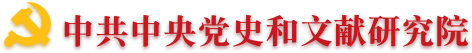•• 理論中國網   |
•"黨的文獻"公眾號"黃城根下"公眾號"黨的歷史"公眾號
•（微博）黨史網
“紀念”是篇大文章

“紀念”是篇大文章

1975629，鄧小平對胡喬木說過這樣一段話：“要利用各種周年紀念寫文章，例如《整頓黨的作風》發表多少周年紀念，這樣寫文章的題就多了。”關於為什麼要重視紀念活動，為什麼要利用周年紀念寫文章，鄧小平沒有展開論述，但這段話卻包含深意。“紀念”是篇大文章，我們利用周年、紀念日等各種場合寫文章、開展紀念活動，對於宣傳愛國主義思想，宣傳中華民族和我們黨的優良傳統，宣傳人類文明和世界發展的進步成果，教育和鼓舞人民、凝聚社會力量，維護國家統一和民族團結，不斷推進中國特色社會主義事業和促進人類進步事業，有著重要的意義。

〔作者孫業禮，中共中央文獻研究室編審，北京100017

（來源：《黨的文獻》2012年第1期）

### 專家學者

• 翻譯
• C
• D
• F
• G
• H
• J
• L
• M
• O
• P
• Q
• S
• T
• W
• X
• Y
• Z
• C
• F
• G
• H
• J
• L
• P
• Q
• S
• T
• W
• X
• Y
• Z
• C
• D
• G
• H
• J
• L
• Q
• S
• W
• X
• Y
• Z